• 首先整体的系统：来源：【图文】狼群智能算法简述_百度文库https://wenku.baidu.com/view/e4f45d6c04a1b0717fd5ddaf.html算法的步骤：公式：上一笔记有记录初始化的数据以及表示：， ， 算法流程图算法收敛性分析：...
首先整体的系统：来源：【图文】狼群智能算法简述_百度文库https://wenku.baidu.com/view/e4f45d6c04a1b0717fd5ddaf.html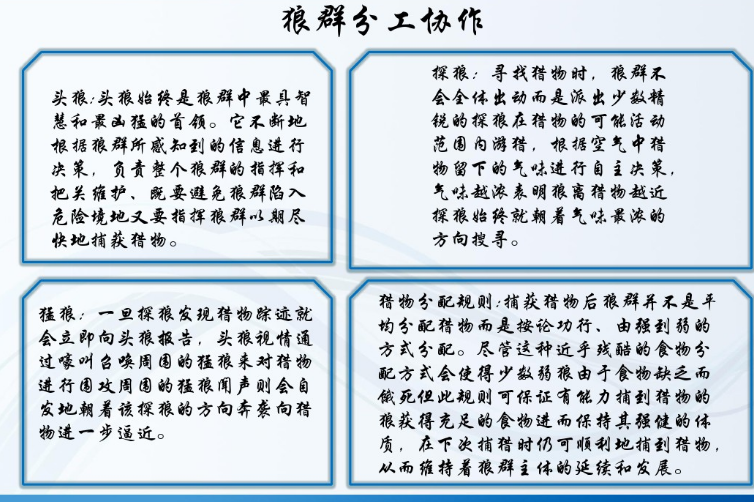算法的步骤：公式：上一笔记有记录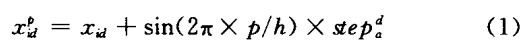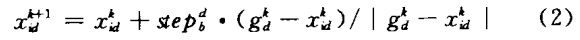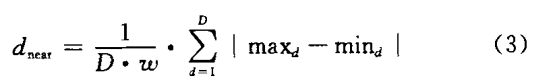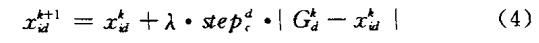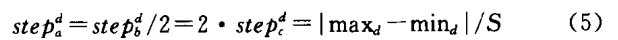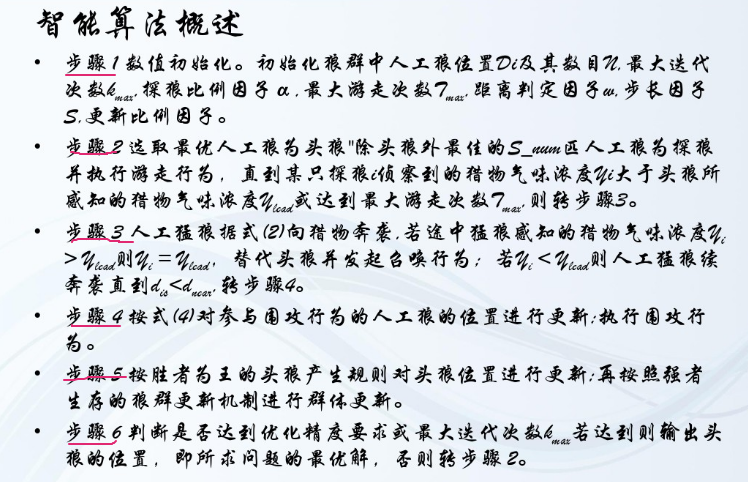初始化的数据以及表示：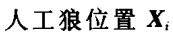，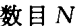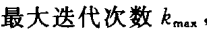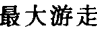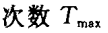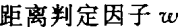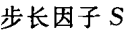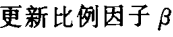，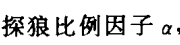算法流程图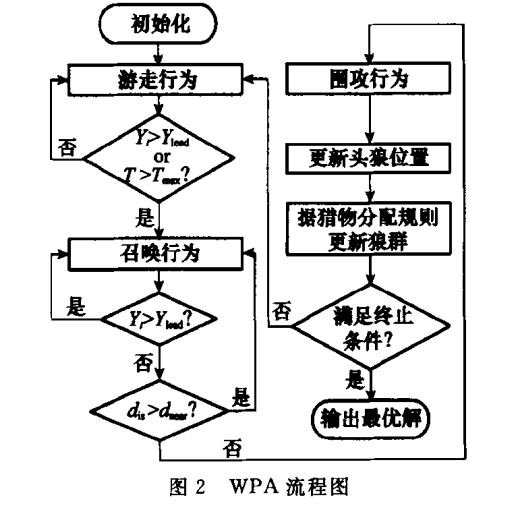算法收敛性分析：使用的是Markov链（一种无后效性的随机过程）无后效性_百度百科https://baike.baidu.com/item/%E6%97%A0%E5%90%8E%E6%95%88%E6%80%A7/1135283?fr=aladdin无后效性原则，指的是这样一种性质：某阶段的状态一旦确定，则此后过程的演变不再受此前各状态及决策的影响。也就是说，“未来与过去无关”，当前的状态是此前历史的一个完整总结，此前的历史只能通过当前的状态去影响过程未来的演变。具体地说，如果一个问题被划分各个阶段之后，阶段k中的状态只能通过阶段k+1中的状态通过状态转移方程得来，与其他状态没有关系，特别是与未发生的状态没有关系，这就是无后效性。  WPA是不断的重复，游走，召唤，围攻的行为的，每种行为只与当前的群体状态有关（当前的第K待更新的群体已经是历史的节点处了。目前的之和节点k+1的新开始记录的历史有关），而与以前的无关。证明的前提：设搜索空间H，游走，召唤，围攻行为会引起状态空间中的状态转移，所以可以使用转移矩阵S，M和W来分别表示他们的影响，则定义的Markov链的转移矩阵：          P=S*M*W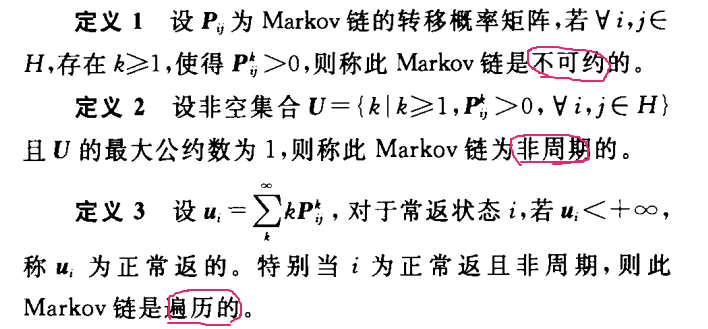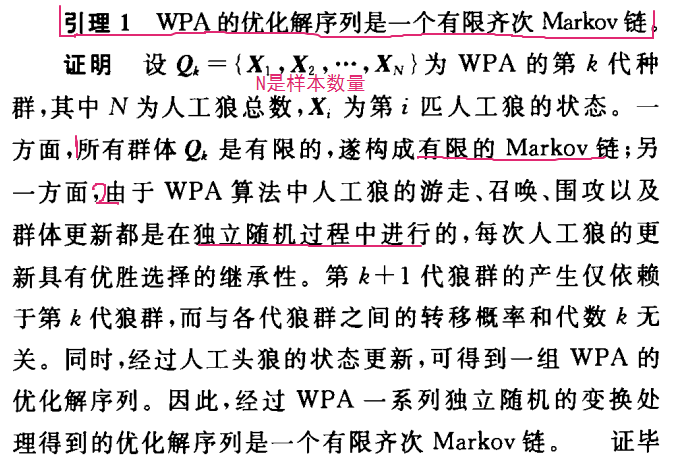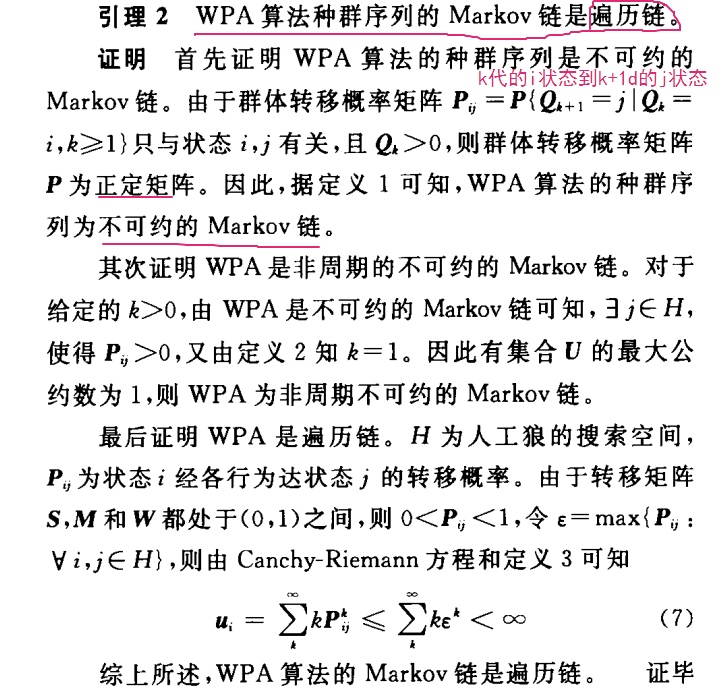正定矩阵_百度百科https://baike.baidu.com/item/%E6%AD%A3%E5%AE%9A%E7%9F%A9%E9%98%B5/11030459?fr=aladdin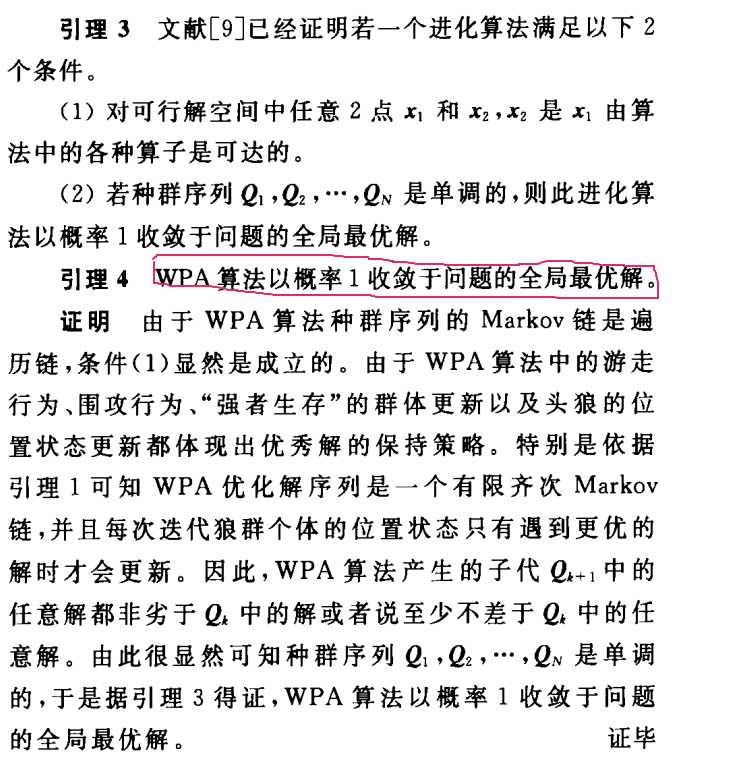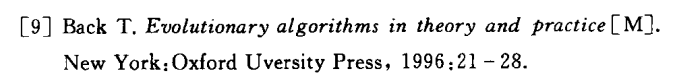展开全文• 确定优化算法和随即优化算法是有明显的分界线的。如果加上分布式集群上的实现方式，就可以分为同步或异步的算法。从梯度下降（GD）后，20世纪50年代，各种一阶算法井喷，其中 SGD 也是这个时候的产物。 梯度下降...
优化算法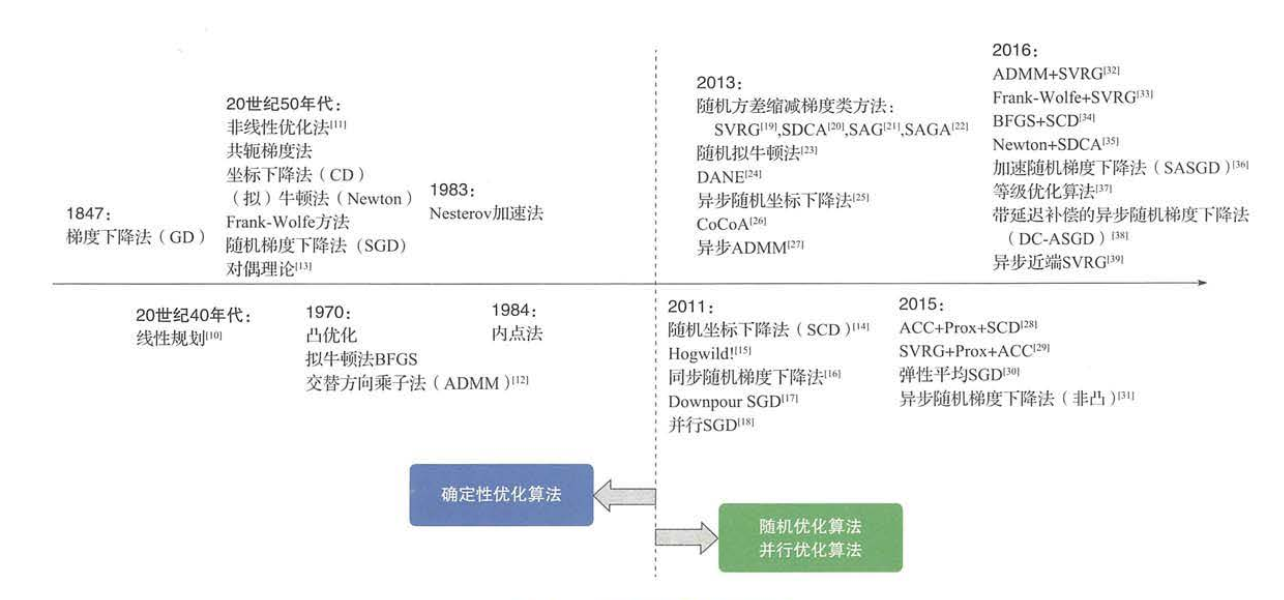上图中，可以看出，确定性优化算法和随即优化算法是有明显的分界线的。如果加上分布式集群上的实现方式，就可以分为同步或异步的算法。从梯度下降（GD）后，20世纪50年代，各种一阶算法井喷，其中 SGD 也是这个时候的产物。
对算法的分析 可以分为一阶的还是二阶的，对偶的还是非对偶的，确定的还是随机的。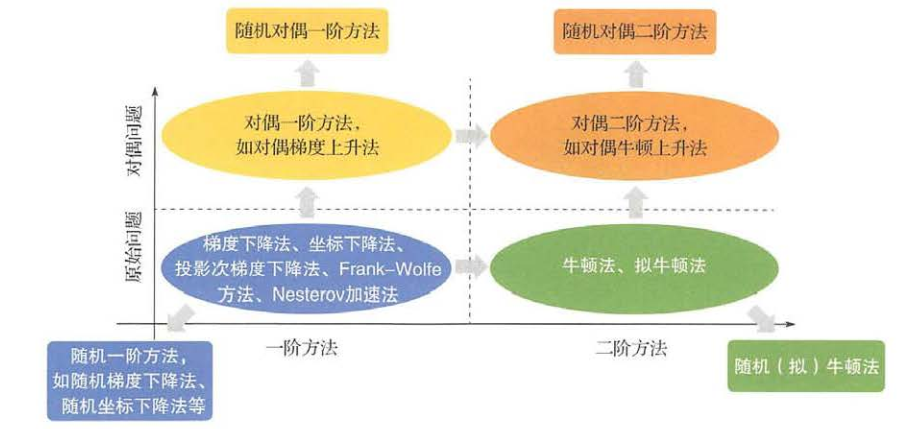梯度下降
梯度下降（GD）是柯西（Cauchy ）大神的1847年提出的。其基本思想是：最小化目标函数在当前状态的一 阶泰勒展开，从而近似地优化目标函数本身 :
$min f(w^*) =min\{ f(w_t) + \nabla f(w_t)^T(w^*-w_t)\} \quad \quad (1)$
假设 $w=w_{t+1}$，因为 $w_t-w_{t+1}$ 是一个小的矢量，因为太大的话（1）式就不成立了（泰勒公式），注意，这里 $f(w_t)$ 是已知的。假设 $w_t-w_{t+1}=\eta v$，其中 $v$ 是单位向量，因为$w_{t+1}$的方向不确定，这里$v$的方向也式不确定的（即我们确定了$v$，$w_{t+1}$ 的方向也就确定了）。于是，（1）式可以表示为：
$min f(w_{t+1}) = min f(w_t) +min \nabla \eta v f(w_t)^T \quad \quad (2)$
这里，我们需要让 $f(w_{t+1}) = f(w_t)<0$（最小化目标函数，也是梯度下降的目的），$\eta>0$ 的小的常数，于是，得到：
$v\nabla f(w_t)^T<0 \quad \quad (3)$
我们需要最小化（3）式。这里，求两个向量的乘积小于零，代表其方向相反，为使得（2）式右端最小，可以取 $v$ 为：
$v=-\frac{\nabla f(w_t)}{||f(w_t)||} \quad \quad (4)$
（上式可根据：$A\cdot B=||A|| \cdot ||B|| cos\alpha$，取$cos \alpha=-1$）
这里，将（4）式中的 $||\nabla f(w_t)||$ 合并到常数项 $\eta$ 中。根据 $w_t-w_{t+1}=\eta v$，于是得到梯度下降法的更新规则如下：
$w_{t+1}=w_{t}-\eta \nabla f(w_t) \quad \quad (5)$
在推出梯度下降规则（5）的过程中，用到了假设 $w_t-w_{t+1}=\eta v$，这个假设其实有某些限制条件，即 $w_t$ 的所有分量都在 $v$ 方向上减少 $\eta$ 得到 $w_{t+1}$，即限制了 $w_{t+1}$ 的空间，即只能在某个方向上变动，因为梯度下降是一维曲线，这样的假设是合理的。还有，在这里将 $||\nabla f(w_t)||$ 合并到常数项 $\eta$ 中有个问题，$||\nabla f(w_t)||$ 是梯度的范数，它是变化的，合并后我们还使用固定常数 $\eta$，就造成了（5）式不严格成立。所以，常用的梯度下降也是变步长的！ 因为没有归一化梯度，这其实符合实际，因为梯度大时，就多走一段距离，小时就少走一点。其实，关于 $\eta$ 真的有好多工作要做！这也是一系列自适应算法的由来。
将其代入上式，得：
$f(w_t)-f(w_{t+1})=\eta \nabla f(w_t)^T \nabla f(w_t) \quad \quad (6)$
这里，得到一个奇怪的结论，因为等式（3）右边是梯度的内积乘以一个常数，所以是大于零的。所以得到：$f(w_t)-f(w_{t+1})>0$ 一定成立，也就是使用梯度下降法梯度一定是下降的！为什么会这样呢？//TODO 想明白了再解决这些谬论。
收敛性分析
对于（5）式中的 $\eta$，我们要做一些限制，从而使得目标函数（1）式是收敛的。
关于函数的各种性质的定义参见 机器学习之优化算法（一）之损失函数，这篇文章里有函数性质（Lipschitz连续、凸、光滑）的定义。
收敛性
这里用变量 $x$ 代替参数 $w$。这里，考察第 t 步迭代 $x_t$ 与 $x^*$ 的距离。
假设目标函数 $f$ 是 $R^d$ 上的凸函数，并且$\beta-$光滑。当步长 $η=\frac{1}{\beta}$ 时，梯度下降法是收敛的。
根据梯度下降公式（5）有：
$||x_{t+1}-x^*||^2=||x_t-\eta\nabla f(x_t)-x^*||^2 \quad \quad (7)$
等式右边开平方得：
$||x_{t+1}-x^*||^2=||x_t-x^*||^2 - 2\eta\nabla f(x_t)^T(x_t-x^*)+\eta^2||\nabla f(x_t)||^2 \quad \quad (8)$
根据$\beta-$平滑性质2有：
$f(x_t)-f(x^*) \leq \nabla f(x_t)^T(x_t-x^*)-\frac{1}{2\beta}||\nabla f(x_t)-\nabla f(x^*)||^2 \quad \quad (9)$
因为$x^*$为最终解，$\nabla f(x^*)=0, f(x_t)>f(x^*)$，代入（9）式得到：
$-\nabla f(x_t)^T(x_t-x^*) \leq -\frac{1}{2\beta}||\nabla f(x_t)||^2 \quad \quad (10)$
将（10）代入（8）得到：
$||x_{t+1}-x^*||^2 \leq ||x_t-x^*||^2-\frac{\eta}{\beta}||\nabla f(x_t)||^2 + \eta^2||\nabla f(x_t)||^2$
$=||x_t-x^*||^2-\eta\left(\frac{1}{\beta}-\eta\right)||\nabla f(x_t)||^2 \quad \quad (11)$
所以，当 $\eta <\frac{1}{\beta}$ 时，上式是收敛的。
最优解
这里，考察第 t 步迭代 $f(x_t)$ 与 $f(x^*)$ 的距离，我们要最小化这个距离，即损失最小。
由$\beta-$光滑性质1，有：
$f(x_{t+1})-f(x_t)\leq \nabla f(x_t)^T(f(x_{t+1})-f(x_t))+\frac{\beta}{2}||x_{t+1}-x_t||^2$
$=-\eta||\nabla f(x_t)||^2-\frac{\beta}{2}\eta^2||\nabla f(x_t)||^2=-\eta(1-\frac{\beta\eta}{2})||\nabla f(x_t)||^2 \quad \quad (12)$
将$f(x^*)$ 代入（12）得：
$[f(x_{t+1})-f(x^*)]\leq [f(x_t)-f(x^*)]-\eta(1-\frac{\beta\eta}{2})||\nabla f(x_t)||^2 \quad \quad (13)$
根据凸函数的性质，有：
$f(x_{t})-f(x^*)\leq \nabla f(x_t)^T(x_t-x^*) \leq ||\nabla f(x_n)||\cdot ||x_n-x^*||$，即：
$-||\nabla f(x_t)|| \leq -\frac{f(x_{t})-f(x^*)}{||x_t-x^*||} \quad \quad (14)$
将（14）代入（13）得：
$[f(x_{t+1})-f(x^*)]\leq [f(x_t)-f(x^*)]-\eta(1-\frac{\beta\eta}{2})\frac{[f(x_{t})-f(x^*)]^2}{||x_t-x^*||^2} \quad \quad (15)$
两边同除 $[f(x_{t+1})-f(x^*)][f(x_t)-f(x^*)]$ 得：
$\frac{1}{f(x_t)-f(x^*)} \leq \frac{1}{f(x_{t+1})-f(x^*)}+\frac{\eta(1-\frac{\beta\eta}{2})}{||x_0-x^*||^2}\frac{f(x_{t})-f(x^*)}{f(x_{t+1})-f(x^*)} \quad \quad (16)$
由$\frac{f(x_{t})-f(x^*)}{f(x_{t+1})-f(x^*)}>1$，并由$||x_0-x^*||^2>||x_t-x^*||^2$替换掉 $x_t$ 得：
$\frac{1}{f(x_t)-f(x^*)} \leq \frac{1}{f(x_{t+1})-f(x^*)}+\frac{\eta(1-\frac{\beta\eta}{2})}{||x_0-x^*||^2} \quad \quad (17)$
对（17）从 0 累加到 T-1 得：
$\frac{1}{f(x_t)-f(x^*)}-\frac{1}{f(x_0)-f(x^*)} \geq \frac{1}{||x_0-x^*||^2} t \eta(1-\frac{\beta\eta}{2}) \quad \quad (18)$
左边第二项是正数：
$\frac{1}{f(x_t)-f(x^*)} \geq \frac{1}{||x_0-x^*||^2} t \eta(1-\frac{\beta\eta}{2})$，即：
$f(x_t)-f(x^*) \leq ||x_0-x^*||^2\cdot \frac{1}{\eta(1-\frac{\beta\eta}{2})} \cdot \frac{1}{t} \quad \quad (19-1)$
如果，每步的 $\eta$ 不同，设为 $\eta_t$，这里（19）就变成了：
$f(x_t)-f(x^*) \leq ||x_0-x^*||^2\cdot \frac{1}{\sum_{t=0}^{t=n-1} \eta_t(1-\frac{\beta\eta_t}{2})} \cdot \quad \quad (19-2)$
这里，我们需要计算一个级数 $\sum_{t=0}^{t=n-1} \eta_t(1-\frac{\beta\eta_t}{2})$ 的收敛性分析了，而且这个级数越大越好，分析见推论2。
这里，我们使用（19-1），那么我们希望使得（19-1）取最小（损失最小），即 $\eta(1-\frac{\beta\eta}{2})$，取最大值，即 $\eta=\frac{1}{\beta}$ 时，可以使得总体loss最小。
推论1：由（19-2）得：
$f(x_t)-f(x^*) \leq \frac{ 2\beta||x_0 - x^*||^2}{ t-1} \quad \quad (20)$
该算法的收敛率为 $\Theta(1/T)$。
推论2：由（19-1），假设 $\eta_t$ 满足 $\sum_{t=1}^{t=T}\eta_t=\infty$，而且  $\sum_{t=1}^{t=T} {\eta_t}^2=\infty$。那么，梯度下降可以收敛到全局最优点。当级数 $\eta_t=1/t$ 时，注意，该级数时发散的（$\sum_{t=1}^{t=T}1/k=\infty$）！
$f(x_t)-f(x^*) \leq \Theta\left(\frac{1}{\log(t)}\right){|| x_0 - x^*||^2} \quad \quad (21)$
可以看出，随着步长 $\eta_t$ 减少，我们可以不对 $\beta$ 进行要求，而且 $\sum_{t=1}^{t=T} {\eta_t}^2=\infty$ 也只是充分不必要的。这里，我们需要 级数 $\sum_{t=0}^{t=n-1} \eta_t(1-\frac{\beta\eta_t}{2})$ 对于 t 是定义良好的。例如：当 $\eta_t=1/log(t)$ 时， 可以得到 (by approximating the sum by a Riemannian integral)：
$\displaystyle \sum_{t=0}^{T-1} \eta_t\left( 1-\frac{\beta \eta_t}{2}\right) \sim \frac{\beta T}{2\log(T)} \quad \quad (22)$
由（19-2），得到：
$\displaystyle f(x_n)-f(x^*) \preceq \frac{2\log(n) || x_0 - x^*|| ^2}{ \beta n} \quad \quad (23)$
这样，就产生了 $log(T)$ 的收敛率，而不是 $1/T$，如下图：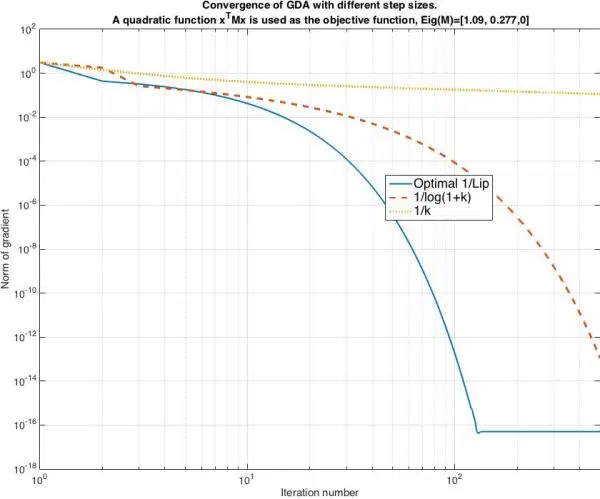总步数
这里，我们假设优化算法从 $x_0$ 开始，而且损失依赖于初始点和最优点之间的距离，假设两点之间的距离半径为 $R$。当 $\eta=1/\beta$ 时，将得到：
$\displaystyle \begin{array}{rcl} f(x_n) -f(x^*) &\leq & \frac{2\beta R ^2}{(n-1)} \le \epsilon \end{array}$
$\displaystyle \begin{array}{rcl} \epsilon &\geq & \frac{2\beta R ^2}{(n-1)}\\ n-1 &\geq & \frac{2\beta R ^2}{}\\ n &\geq & \frac{2\beta R ^2}{\epsilon}\end{array} \quad \quad (24)$
（24）表明最小步长为 $\frac{2\beta R^2}{\epsilon}$ ，而该结果的收敛性直接依赖 Lipschiz 常数 $\beta$、据初始点的距离和容忍集是否可逆。
注意：

Lipschiz 连续一般都假设在 凸优化的基础上。
$\beta-$光滑，凸都不能单独保证有好的收敛率，而 $\alpha-$强凸则可以保证有较快的收敛率。
梯度下降算法和数据的维度有线性关系。

$\alpha-$强凸
最优值
引理1：如果 f 即是 ${\beta-}$光滑 又是 ${\alpha}-$强凸. 对于 ${\forall x,y \in {\mathbb R}^n}$，
$\displaystyle \begin{array}{rcl} (\nabla f(x) -\nabla f(y))^T \geq \frac{\alpha\beta ||x-y||^2}{\alpha + \beta} + \frac{||\nabla f(x) - \nabla f(y)||^2}{\alpha +\beta}. \end{array} \quad \quad (25)$
定理1：假设 ${f}$ 是 ${\beta}$-光滑和${\alpha}$-强凸函数。 那么，GD的步长 ${\eta_t \leq 2/(\alpha+\beta)}$ 满足：
$\displaystyle f \left( x_t \right) - p^* \leq \frac{\beta}{2} \prod_{t=1}^T \left( 1 - \frac{2\eta_t \alpha \beta}{\alpha + \beta} \right) \Vert x_0 - x^* \Vert^2 \quad \quad (26)$
证明：将公式梯度下降公式（5）代入 ${\beta}$-光滑的性质（1）（参考这里）式 $|f(x)-f(y)-\nabla f(y)^T(x-y) | \leq \frac{\beta}{2}||x-y||^2$ 的条件中，得：
$\displaystyle f \left( x_k \right) \leq f \left( x^* \right) + \nabla f \left( x^* \right)^T \left( x_k - x^* \right) + \frac{\beta}{2} \Vert x_k - x^* \Vert ^2 \quad \quad (27)$
根据最优点的梯度 $\nabla f(x^*)=0$，则(27) 得：
$\displaystyle f \left( x_k \right) - f \left( x^* \right) \leq \frac{\beta}{2} \Vert x_k -x^* \Vert ^2 \ \ \ \ \ (28)$
${\Vert x_{t+1} - x^* \Vert^2}$ 服从等式（8），即:
$\displaystyle \begin{array}{rcl} \Vert x_{t+1} - x^* \Vert^2 = \Vert x_t - x^* \Vert^2 + \eta_t^2 \Vert \nabla f \left( x_t \right) \Vert^2 -2\eta_t \nabla f \left( x_t \right)^T\left( x_t - x* \right) \end{array} \quad \quad (8-2)$
使用引理等式（25）和 $\nabla f(x^*)=0$ 得：
$\displaystyle \Vert x_{t+1} - x^* \Vert^2 \leq \Vert x_t - x^* \Vert^2 + \eta_t^2 \Vert \nabla f \left( x_t \right) \Vert^2 -2\eta_t \left( \frac{\alpha \beta}{\alpha + \beta} \Vert x_t - x^* \Vert^2 + \frac{\Vert \nabla f \left( x_t \right) \Vert^2}{\alpha + \beta} \right) \quad \quad (29)$
化简得：
$\displaystyle \begin{array}{rcl} \Vert x_{t+1} - x^* \Vert^2 \leq& \left( 1 - 2\eta_t \frac{\alpha \beta}{\alpha + \beta} \right) \Vert x_t - x^* \Vert^2 + \eta_t \left( \eta_t - \frac{2}{\alpha + \beta} \right) \Vert\nabla f \left( x_t \right) \Vert^2 \end{array} \quad \quad (30)$
因为 ${\eta_t <\frac{2}{\alpha + \beta}}$，将右手边（RHS）得最后一项忽略，可以得到：
$\displaystyle \Vert x_{t+1} - x^* \Vert^2 \leq\left( 1 - 2\eta_t \frac{\alpha \beta}{\alpha + \beta} \right) \Vert x_t - x^* \Vert^2 \quad \quad (31)$
对 $t$ 进行迭代，得：
$\displaystyle \Vert x_t - x^* \Vert ^2 \leq \Vert x_0 - x^* \Vert ^2 \prod_{t=1}^T \left( 1 - \frac{2\eta_t \alpha \beta}{\alpha + \beta} \right) \quad \quad (32)$
将式（32）代入 $\beta-$ 光滑条件 (28)，可得式（26），定理得证。
收敛率
根据 ${(1-x) \leq \exp (-x)}$，定理1 可以改写为下式：
$\displaystyle f \left( x_k \right) - p^* \leq \frac{\beta}{2} \Vert x_0 - x^* \Vert^2 e^{-\frac{2\alpha\beta}{\alpha+\beta}\sum_{t=1}^T \eta_t} \ \ \ \ \ \ \ \ \ \ (33)$
引理2：如果GD算法得步长为 $\eta=\frac{2}{\alpha+\beta}$ ，在${\beta-}$光滑 又是 ${\alpha}-$强凸条件下，满足：
$\displaystyle \begin{array}{rcl} f \left( x_t \right) - p^* &\leq \frac{\beta}{2} \left( \frac{Q_f - 1}{Q_f + 1} \right)^{2t} \Vert x_0 - x^* \Vert^2 \\ &\leq \frac{\beta}{2} \exp \left( - \frac{4t}{Q_f+1} \right) \Vert x_0 - x^* \Vert^2 \end{array} \ \ \ \ \ \ (34)$
这里，${Q_f = \frac{\beta}{\alpha}}$ 为条件数。
证明：将 $\eta=\frac{2}{\alpha+\beta}$ 代入定理1，式（26），得：
$\displaystyle \begin{array}{rcl} f \left( x_t \right) - p^* &\leq \frac{\beta}{2} \prod_{t=1}^T \left( 1 - \frac{2\eta_t \alpha \beta}{\alpha + \beta} \right) \Vert x_0 - x^* \Vert^2 \\ &= \frac{\beta}{2} \left( 1 - \frac{2}{Q_f+1} \right)^{2t} \Vert x_0 - x^* \Vert^2 \\ & \leq \frac{\beta}{2} \exp \left( \frac{-4t}{Q_f+1} \right) \Vert x_0 - x^* \Vert^2 \end{array} \ \ \ \ \ \ (35)$
得证。引理2说明在强凸和固定步长下，GD可以取得指数级得收敛率。但在凸分析中，$\exp (-x)$是线性收敛率（linear convergence），$\exp (\exp(-x))$ 是二次收敛率（quadratic convergence）。可以看出，收敛率取决于条件数 ${Q_f = \frac{\beta}{\alpha}}$：大的条件数有小的收敛率。
逐步缩小步长
引理3：$\eta_t，其他条件不变，得：
$\displaystyle f(x_t)- p^* \leq \frac{\beta}{2 t^{\frac{2c\alpha\beta}{\alpha+\beta}}}\|x_0 -x^*\|^2 \quad \quad (36)$
证明：将 代入式（33），根据 ${\sum_{t=1}^T 1/t \sim \log(t)}$，可得：
$\displaystyle f \left( x_t \right) - p^* \leq \frac{\beta}{2} \Vert x_0 - x^* \Vert^2 e^{-\frac{2c\alpha\beta}{\alpha+\beta} \log(t)} \quad \quad (37)$
由（37）可得（36）。
该引理说明，随着 $c$ 的增加，GDA可以取得多项式的收敛率。但对于太大的 $c$，在初始阶段可能违反 $\eta_t<\frac{2}{\alpha+\beta}$。尽管算法在初始阶段可能会变慢，但对于大的$t$，收敛率仍然可以由引理（3）给出：${\frac{c}{k} < 2/(\alpha+\beta)}$。

当目标函数是强凸函数时，梯度下降法的收敛速率是线性的;当目标函数是凸函数时，其收敛速率是次线性的 。 也就是说，强凸性质会大大提高梯度下降法的收敛速率 。 进一步地，强凸性质越好(即 $\alpha$ 越大) ，条件数 $Q$ 越小，收敛越快 。
光滑性质在凸和强凸两种情形下都会加快梯度下降法的收敛速率，即 $\beta$ 越小(强凸情形下，条件数 $Q$ 越小 ) ，收敛越快。

这里，只分析了 GD 的收敛性，并没有分析在仅仅 Lipschitz 连续 (非凸) 下的情况，需要将梯度下降等式（5）代入 Lipschitz 连续的条件，然后逐项相加，分析T步的收敛情况，得到关于步长 $\eta_t$ 的级数，通过对该级数分析，就可以得到其最好最坏的收敛率，这种情况下的收敛率大概是次线性的（subliner convergence）。
其实，收敛率是由 $f$ 决定的，但对 $\eta_t$  级数的设计和修改可以加快收敛速度。

参考自这里。


展开全文优化 优化算法 梯度下降 机器学习
• 一致有界性原理的一个应用就是序列和算子的收敛性分析。 文章目录1. 序列收敛性2. 线性泛函收敛性3. 一般有界线性算子收敛性4. 应用举例 1. 序列收敛性 (X,∥⋅∥)(X,\Vert\cdot\Vert)(X,∥⋅∥)，有 xn,x∈Xx_n,x\...
一致有界性原理的一个应用就是序列和算子的收敛性分析。
文章目录1. 序列收敛性2. 线性泛函收敛性3. 一般有界线性算子收敛性4. 应用举例
1. 序列收敛性
$(X,\Vert\cdot\Vert)$，有 $x_n,x\in X$，称 $x_n$ 强收敛到 $x$，若 $\Vert x_n-x\Vert \to 0$；称 $x_n$ 弱收敛到 $x$ 若 $\forall f\in X'$ 都有 $f(x_n)\to f(x)$，记为 $x_n \stackrel{w}{\longrightarrow} x.$
关于弱收敛有以下几条性质：

若 $x_n \stackrel{w}{\longrightarrow} x, x_n \stackrel{w}{\longrightarrow} y$，则 $x=y$；
若 $x_n \stackrel{w}{\longrightarrow} x$，则存在 $c\ge0, \Vert x_n\Vert \le c.$

证明：仅证第二条。这个性质说明 $x_n$ 有界，因此容易想到需要用一致有界性原理证明，但是该原理说明的是算子的一致有界，这里是元素 $x_n$ 有界，因此又可以想到上一篇讲到的典范映射 $J:X\to X''$ 从元素映射到算子。因此这里考虑 $X'$ 上的线性泛函 $g_n= J(x_n):X'\to \mathbb{R}$，有 $g_n(f)=f(x_n),\forall f\in X'.$ 于是有 $f(x_n)\to f(x)$，因而固定任一 $f$，都有 $\sup_n g_n(f) < \infty$，同时由于 $X'$ 总为 Banach 空间，利用一致有界性原理有 $\sup_n \Vert g_n\Vert =\sup_n \Vert x_n\Vert < \infty$。证毕。

定理：$(X,\Vert\cdot\Vert)$，有 $x_n,x\in X$，则 $x_n \stackrel{w}{\longrightarrow} x$ 当且仅当：

存在 $c\ge0,\Vert x_n\Vert\le c$；
并且存在 $M\subset X',\overline{\text{span}M}=X'$，对 $\forall f\in M, f(x_n)\to f(x).$（此时 $M$ 称为完全集）

NOTE：该定理简化了弱收敛的判断条件，只需要在 $X'$ 的一个子集上判断函数值是否收敛。

证明：$"\Longrightarrow"$ 易证；
$"\Longleftarrow"$，首先考虑 $\forall f\in \text{span}M$，容易得到 $f(x_n)\to f(x)$。然后对 $\forall g\in X'$，那么存在 $f_m\in\text{span}M$ 使得 $\Vert f_m-g\Vert \le 1/m$，因此
KaTeX parse error: No such environment: align at position 8:
\begin{̲a̲l̲i̲g̲n̲}̲
|g(x_n)-g(x)|&…
证毕。
例子 1：考虑 $X=\ell^p(1，有 $(\ell^p)'=\ell^q, 1/p+1/q=1.$ 考虑线性泛函 $f_y(x)=\sum_i y_ix_i,y\in \ell^q$，有 $\Vert f_y\Vert=\Vert y\Vert_q$。我们考虑 $X'$ 的子空间 $M=\{e_n,n\ge1\}$，其中 $e_n=(...,0,1,0,...)$ 表示只有第 $n$ 个分量为 1，其余为 0。那么 $\overline{\text{span}M}=X'$，因此要想验证 $x_n$ 是否弱收敛到 $x$ 就只需要验证：1）其有界性；2）对每个 $f_{e_k},k\ge1$ 是否有 $f_{e_k}(x_n)\to f_{e_k}(x)(n\to\infty).$
强收敛与弱收敛之间有如下关系：

$x_n\to x \Longrightarrow x_n \stackrel{w}{\longrightarrow} x$(即强收敛可以导出弱收敛)；
若 $\text{dim}X<\infty$，则 $x_n \stackrel{w}{\longrightarrow} x\Longrightarrow x_n\to x$(有限维赋范空间中，强收敛与弱收敛等价)；

证明：仅证第二条。设 $\text{dim}X=n<\infty$，有限维赋范空间中我们可以找到一组基，$x_k=\lambda_{k,1}e_1+\cdots+\lambda_{k,n}e_n$，$x=\lambda_1 e_1+\cdots+\lambda_n e_n$。那么
$\lambda_{k,1}f(e_1)+\cdots+\lambda_{k,n}f(e_n) \to \lambda_{1}f(e_1)+\cdots+\lambda_{n}f(e_n), \quad\forall f\in X'$
由于 $f\in X'$ 任取，那么我们可以取 $f_i(y)=\mu_i$，其中 $y=\mu_1 e_1+\cdots+\mu_n e_n$，即 $f_i$ 取出来第 $i$ 个坐标系数。由此可以得到 $\lambda_{k,i}\to\lambda_i(k\to\infty)$，然后就容易得到 $x_n\to x.$ 证毕。
例子 2：有些无穷维空间中也可以得到 $x_n \stackrel{w}{\longrightarrow} x\iff x_n\to x$，例如 $\ell^1.$
例子 3：无穷维 Hilbert 空间（$\ell^2$，注意只有 $2-$范数才能定义出对应的内积），考虑 $\{e_1,e_2,\ldots\}$ 为 $H$ 的标准正交集，那么有 $e_n \stackrel{w}{\longrightarrow} 0$ 但是 $e_n \nrightarrow 0$。考虑 $\forall f\in H'$，存在唯一的 $z_0\in H, f(x)=\langle x,z_0\rangle$，由Bessel方程 $\sum_n|\langle e_n,z_0\rangle|^2\le \Vert z_0\Vert^2$，因此 $f(e_n)\to 0(n\to \infty),\forall f\in H'$，但另一方面 $\Vert e_n\Vert=1\nrightarrow0$。
2. 线性泛函收敛性
对于算子的收敛性，如线性泛函 $f_n\in X'$ 或者有界线性算子 $T\in B(X,Y)$，收敛性的定义跟上面序列的收敛性是相似的，但是又略有不同。下面就先给出线性泛函收敛性的分析。
同样考虑赋范空间 $X$，$f,f_n\in X'$，称 $f_n$ 弱星收敛到 $f$，若任取 $x\in X$ 都有 $f_n(x)\to f(x)$，记为 $f_n \stackrel{w\star}{\longrightarrow} f.$
NOTE：实际上这里的弱星收敛跟序列的弱收敛是完全对称的，因此他们的性质也是类似的。

弱星收敛极限 $f$ 唯一；
$\{f_n\}$ 的任意子列均弱星收敛到 $f$；
若 $X$ 为 Banach 空间，则 $\{f_n\}$ 在 $X'$ 中为有界集。

证明：仅证第三条，对于任意 $x\in X$，有 $f_n(x)\to f(x)$，因此 $f_n(x)$ 有界，由一致有界性原理，$\sup_n \Vert f_n\Vert<\infty.$ 证毕。

定理：$X$ 为 Banach 空间，$f_n,f\in X'$，则 $f_n \stackrel{w\star}{\longrightarrow} f$ 当且仅当：

存在 $c\ge0,\Vert f_n\Vert\le c$；
并且存在 $M\subset X,\overline{\text{span}M}=X$，对 $\forall x\in M, f_n(x)\to f(x).$

NOTE：该性质与序列弱收敛的性质完全对称，证明省略。

NOTE：对于 $X'$ 中的线性算子 $f$，也有范数的定义，因此我们也可以按照序列的收敛性来定义算子的收敛性。这个时候就用 $X'$ 代替上面的 $X$，用 $X''$ 代替上面的 $X'$。我们可以得到什么样的强收敛和弱收敛定义呢？（下面并不是标准的数学定义，只是我为了引出之后的内容做的解释）
对于 $f,f_n\in X'$，若满足 $\Vert f_n-f\Vert\to 0$，则称 $f_n$ 一致收敛到 $f$；若对 $\forall g\in X''$，都有 $g(f_n)\to g(f)$，那么称 $f_n$ 强收敛到 $f$；弱收敛的定义暂且不管。
注意从这个定义的字面意思来看，这里的一致收敛对应于上面序列的强收敛；这里的强收敛对应上面序列的弱收敛，它实际上也就对应于弱星收敛。这里就有两个值得思考的问题：1）**一致收敛和强收敛的区别是什么？**2）这里的强收敛为什么对应上面的弱收敛？
先看第2个问题：讲 Hahn-Banach 定理应用的时候我们讲到了典范映射，如果 $X$ 为自反的，那么任意一个 $g_0\in X''$ 都唯一的对应于 $X$ 中的元素 $x_0$，并且满足 $g_0(f)=f(x_0),\forall f\in X'$。假设 $X$ 是自反的，那么上面的强收敛定义就可以表述为 $\forall x\in X$，都有 $f_n(x)\to f(x)$，注意看！这是不是就是线性泛函弱星收敛的定义！也对应了序列的弱收敛。不过弱星收敛的定义里面并没有要求 $X$ 是自反的。
那么再看第1个问题：一致收敛中要求 $\Vert f_n-f\Vert\to0$，线性算子的范数是针对整个源空间考虑的；而强收敛中对每个 $x\in X$，关注 $f_n(x)\to f(x)$，也就是说关注的是每一个局部点。因此一致收敛要强于强收敛。

3. 一般有界线性算子收敛性
实际上一致收敛、强收敛、弱收敛的概念可以扩展到任意的有界线性算子定义。
设 $X,Y$ 为赋范空间，$T_n\in B(X,Y),T:X\to Y$ 为线性算子，有三种收敛性：

$\{T_n\}$ 一致收敛到 $T$，若 $\Vert T_n-T\Vert \to 0$；
$\{T_n\}$ 强收敛到 $T$，若 $\forall x\in X,T_n x\to Tx$；
$\{T_n\}$ 弱收敛到 $T$，若任取 $x\in X, f\in Y'$，$f(T_nx)\to f(Tx)$。

容易看出来一致收敛 $\Longrightarrow$ 强收敛 $\Longrightarrow$ 弱收敛，但是反向则不成立，可以举出对应的反例。
例子 4(强收敛 $\nRightarrow$ 一致收敛)：$X=Y=\ell^2$，$T_n:\ell^2\to\ell^2$ 有
$T_n: (x_1,x_2,\cdots) \mapsto (0,\cdots,0,x_{n+1},x_{n+2},\cdots)$
容易验证 $T_n$ 为有界线性算子，$\Vert T_n\Vert=1$。可以验证 $T_n$ 强收敛到 $0$ 算子，即 $T_0x\equiv 0$。但是 $\Vert T_n-T_0\Vert=1\nrightarrow 0$，即不满足一致收敛。
例子 5(弱收敛 $\nRightarrow$ 强收敛)：$X=Y=\ell^2$，$T_n:\ell^2\to\ell^2$ 有
$T_n:(x_1,x_2,\cdots)\mapsto(0_1,\cdots,0_n,x_1,x_2,\cdots)$
可以验证 $T_n$ 为有界线性算子，并且 $\Vert T_n\Vert=1$。是否有 $T_n$ 弱收敛到某个 $T$ 呢？考虑任意 $f\in(\ell^2)'$，都存在唯一的 $z\in\ell^2$，$f(x)=\langle x,z\rangle$，所以 $f(T_nx)=x_1\overline{z_{n+1}}+x_2\overline{z_{n+2}}+\cdots$，因此
$|f(T_nx)|\le\sum_{k=1}^\infty |x_k|\cdot|z_{n+k}| \le \Vert x\Vert\left(\sum_{k=n+1}^\infty |z_k|^2\right)^{1/2} \to 0$
所以有 $f(T_nx) \to 0$ 对任意 $f\in (\ell^2)'$ 成立，因此 $f(T_nx)\to f(T_0x)\equiv f(0)=0$。所以 $T_n$ 弱收敛到 $T_0=0$ 算子，但是总有 $\Vert T_nx\Vert=\Vert x\Vert\nrightarrow 0$，因此 $T_nx\nrightarrow T_0x$，即不满足强收敛。
命题：对于一般有界线性算子，若 $T_n$ 一致收敛到 $T$，则 $T$ 也是有界的，这是因为 $\Vert T\Vert\le \Vert T-T_n\Vert+\Vert T_n\Vert \le \infty$；若只能得到 $T_n$ 强收敛到 $T$，那么 $T$ 不一定是有界的。
例子 6(强收敛极限未必有界)：$X=Y=\{(x_n),\exists N,\forall n\ge N, x_n=0 \}$，考虑 $T_n:X\to Y$ 有
\begin{aligned} T_n:& (x_1,x_2,\cdots)\mapsto (x_1,2x_2,\cdots,nx_n,x_{n+1},x_{n+2},\cdots) \\ T:& (x_1,x_2,\cdots)\mapsto (x_1,2x_2,\cdots) \end{aligned}
那么 $\Vert T_n\Vert=n$，取可以验证对于 $\forall x\in X$，$T_nx\to Tx$，即 $T_n$ 强收敛到 $T$，但是 $T$ 不是有界算子。
那么什么情况下可以保证强/弱收敛极限也是有界算子呢？

定理：设 $X$ 为 Banach 空间，$Y$ 为赋范空间，$T_n\in B(X,Y),T:X\to Y$ 为线性算子。设 $T_n$ 弱收敛到 $T$，则 $\sup_{n\ge1}\Vert T_n\Vert < \infty, T\in B(X,Y)$ 并且 $\Vert T\Vert \le \sup_{n\ge1}\Vert T_n\Vert <\infty.$

证明：由于 $T_n$ 弱收敛到 $T$，即 $\forall x\in X,f\in Y'$ 都有 $f(T_nx)\to f(Tx)$，因此有 $T_nx \stackrel{w}{\longrightarrow} Tx$。那么根据序列弱收敛的性质，存在 $c_x$ 满足 $\sup_n \Vert T_nx\Vert \le c_x$，再由一致有界性原理，有 $\sup_n \Vert T_n\Vert < \infty$。
然后考虑 $T$，$\forall x\in X$，由 Hahn-Banach 定理的推论，都存在 $f\in Y',\Vert f\Vert=1$ 满足
$\Vert Tx\Vert=|f(Tx)| = \lim_{n\to\infty} |f(T_nx)| \le \lim_{n\to\infty}\Vert T_nx\Vert$
因此 $\Vert T\Vert\le \sup_n\Vert T_n\Vert.$ 证毕。

定理：设 $X$ 为 Banach 空间，$Y$ 为赋范空间，$T_n,T\in B(X,Y)$，则 $T_n$ 强收敛到 $T$ 当且仅当：

$\sup_n \Vert T_n\Vert < \infty$；
存在 $M\subset X,\overline{\text{span}M}=X$，对 $\forall x\in M, T_n(x)\to T(x).$

NOTE：这跟线性泛函弱星收敛的等价条件是完全一样的，证明省略。

4. 应用举例
例子 7(求积分的数值方法)：考虑实值函数 $x\in C[a,b]$，并赋予无穷范数，那么 $(C[a,b],\Vert\cdot\Vert)$ 为 Banach 空间，求 $\int_a^b x(t)dt.$
既然是在本节举的这个例子，那就要用到算子收敛性。先定义有界线性算子 $f(x)=\int_a^b x(t)dt$，$\Vert f\Vert=b-a$。我们现在的目标就是找一列有界线性泛函 $f_n$ 弱收敛到 $f$。回忆我们在学微积分的时候，往往是用分段的矩形面积求和来逼近积分。在 $[a,b]$ 上取 $n+1$ 个结点 $a=t_{n,0}，再取 $n+1$ 个实数 $a_{n,0},\cdots,a_{n,n}$，令
$f_n(x) = \sum_{k=0}^n a_{n,k} x(t_{n,k})$
$f_n$ 是 $C[a,b]$ 上的线性泛函，并且 $\Vert f_n\Vert \le \sum_{k=0}^n|a_{n,k}|$，另外我们总能够造出一个 $x\in C[a,b]$ 满足 $x(t_{n,k})=\text{sgn}(a_{n,k})$ 并且 $\Vert x\Vert_\infty=1$，此时就有 $f(x)=\sum_{k=0}^n|a_{n,k}|$，于是可以得到 $\Vert f_n\Vert = \sum_{k=0}^n|a_{n,k}|$。现在的问题就是我们能否找到合适的系数 $a_{n,k}$ 使得 $f_n\stackrel{w}{\longrightarrow} f$ ？
这里我们提出一个额外的要求，就是对于次数小于 $n$ 的多项式 $p$，需要 $f_n(p)$ 能获得精确积分结果，即 $f_n(p)=\int_a^b p(t)dt$。由于 $\{1,t,\ldots,t^n\}$ 构成次数小于 $n$ 的多项式空间的 Hamel 基，所以只需要验证对每个基有 $f_n(e_k)= f(e_k)$ 即可。这就要求
$\begin{cases} \begin{matrix} a_{n,0} & + & a_{n,1} & + & \cdots & + & a_{n,n} & = & b-a \\ a_{n,0}t_{n,0} & + & a_{n,1}t_{n,1} & + & \cdots & + & a_{n,n}t_{n,n} & = & \frac{b^2-a^2}{2} \\ & & & & \ldots & \\ a_{n,0}t_{n,0}^n & + & a_{n,1}t_{n,1}^n & + & \cdots & + & a_{n,n}t_{n,n}^n & = & \frac{b^{n+1}-a^{n+1}}{n+1} \end{matrix} \end{cases}$